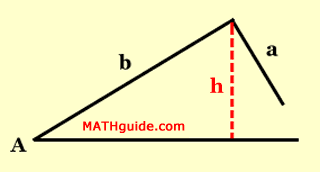Law of Sines: Ambiguous Case (SSA)
View the Lesson | MATHguide homepage Updated July 19th, 2021

Status: Waiting for your responses.Given: A = 37°, a = 12.5, and b = 9
Problem: Calculate the height of the triangle (to the nearest tenth). Then, determine the number of possible triangles by comparing the h, a, and b-values.

 height = Make a selection. 0 triangle 1 triangle 2 triangles